# 10.1016 j.media.2015.08.012 figure

Nov. 23, 2015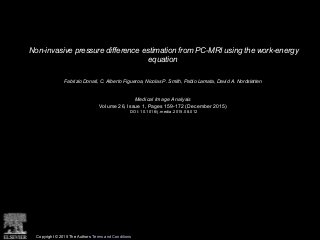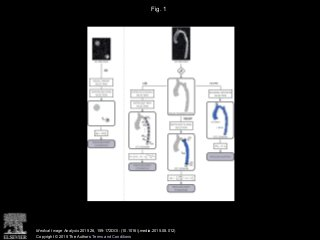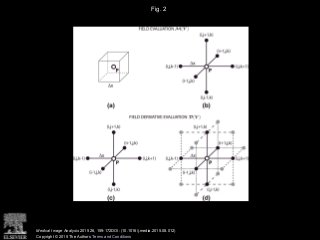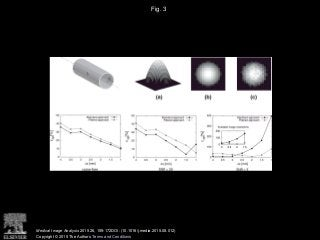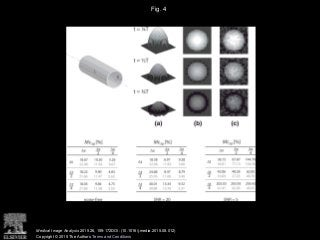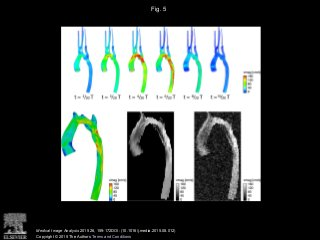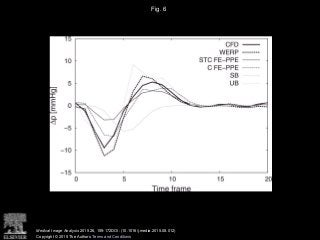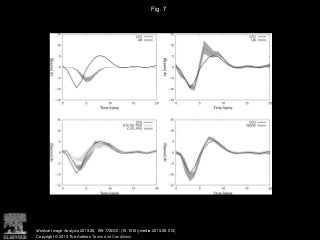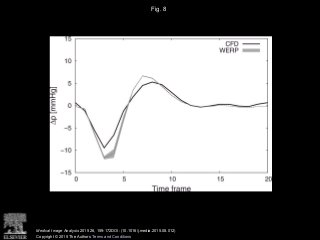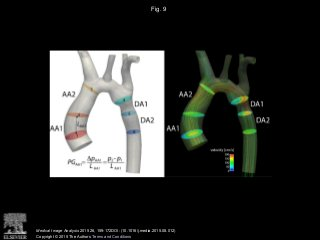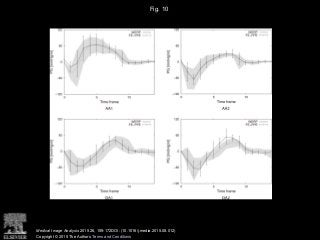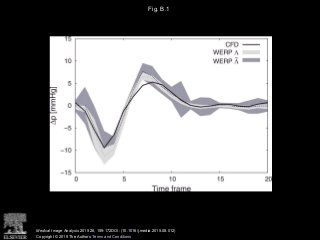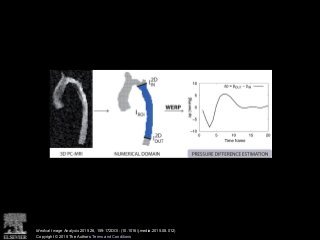1 of 13

### Editor's Notes

1. Schematic representation of the available methods to estimate time-dependent relative pressure non-invasively from PC-MRI, from left to right: Simplified Bernoulli (SB), Unsteady Bernoulli (UB), Work Energy-derived Relative Pressure (WERP), and Finite Element-based Poisson Pressure Equation (FE-PPE) on Core (C) mesh (in blue), and Static Tissue + Core (STC) mesh (in grey).
2. Schematic representation of the finite differences central stencils. Velocity field V evaluation at P=(i,j,k) through operator M(V) using standard (a) and filtered approach (b) and velocity field V derivative evaluation at P=(i,j,k) through operator D(V) using standard (c) and filtered central differences approach (d).
3. Validation of the WERP method on an in silico image data derived from Poiseuille flow. The dimensions of the cylindrical straight phantom (top left) are length L=10cm and radius R=1.5cm. Peak velocity Vmax=1m/s, blood density ρ=1060kg/m3 and dynamic viscosity μ=0.004Pa·s. Top images: visualization of the 3D analytical velocity profile (a), noise-free in-plane image (b) and noisy in-plane image (c). Bottom plots: pressure difference percentage relative error εΔp as a function of image resolution on a noise-free (left), low-noise SNR=20 (center) and high-noise SNR=5 (right) cases; average value over 100 simulation tests. Effect of standard (solid black line) and filtered stencil (solid grey line) with focus on currently available image resolutions.
4. Convergence analysis of the WERP method on an in silico image data derived adding a Womersley and a Poiseuille flow solutions. The dimensions of the cylindrical straight phantom (top left) are length L=10cm and radius R=1.5cm. Peak velocity Vmax=1m/s, blood density ρ=1060kg/m3 and dynamic viscosity μ=0.004Pa·s. Top images: Visualization of the 3D analytical velocity profile at different phases (a), noise-free in-plane image (b) and noisy in-plane image (c). Velocity profiles are shown at different time frames during the simulated cardiac cycle with duration T. Bottom tables: Pressure difference maximum percentage relative error over time MεΔp as a function of space and time resolution, Δx=4mm and Δt=T/8s on a noise-free (left), low-noise SNR=20 (center) and high-noise SNR = 5 (right) cases; average value over 100 simulation tests. Effect of standard (black) and filtered stencil (grey).
5. CFD simulation of a patient-specific model of a human aortic coarctation. Top images: volume-render view of the velocity magnitude field at different phases. Bottom images: axial plane visualizations of the intensity image at time t=6/20T (left), acquired noise-free image with Δx=2mm (centre) and acquired noisy image with Δx=2mm and simulated Gaussian noise with SNR=5 (right).
6. Comparison of pressure differences over the cardiac cycle computed with all available methods against benchmark solution from CFD simulations (solid black line): WERP (dashed black line), STC FE-PPE (solid dark grey line), C FE-PPE (dashed dark grey line), SB (solid light grey line) and UB (dashed light grey line).
7. Sensitivity analysis to noise for the total pressure difference. CFD pressure difference over the cardiac cycle (solid black line) compared against SB (top left), UB (top right), STC FE-PPE (bottom left, light grey), C FE-PPE (bottom left, dark grey) and WERP (bottom right). Average pressure differences computed over 100 simulation tests (dashed lines) and 99% confidence intervals (grey filled curves).
8. Sensitivity analysis to image segmentation process. Comparison of pressure differences over the cardiac cycle computed with WERP against benchmark solution from CFD simulations (solid black line). 99% confidence intervals of pressure differences computed over ROIs extracted using S=20%÷40%V¯max.
9. Definition of the four anatomical regions: ascending aorta - AA1 and AA2 - and descending aorta - DA1 and DA2. Left: illustration of the planes selected to define the anatomical regions. Pressure gradient with WERP method is computed as the pressure difference over a generic region defined by inlet and outlet planes divided by the aortic segment length L. (LAA1 in the example). Right: illustration of velocity magnitude surface plots and velocity streamlines during peak systole.
10. Average temporal profile of the pressure gradient in healthy subjects (n=9) in the four anatomical regions. Variability of the pressure gradients obtained with WERP (grey filled curve) and FE-PPE (errorbar plot) methods.
11. Comparison of pressure differences over the cardiac cycle computed using a boundary flux computed from the outlet plane of the ROI (Λ) or from the outlet and walls surface (Λ˜). CFD pressure difference (solid black line), WERP average pressure difference over 100 simulation tests (dashed black line) and WERP 99% confidence interval (grey filled color).